# ATtiny13 – PI Metal Detector

This experimental project shows how to build an ATtiny13 microcontroller based simple PI (Pulse Induction) metal detector.

IntermediateFull instructions provided2 hours330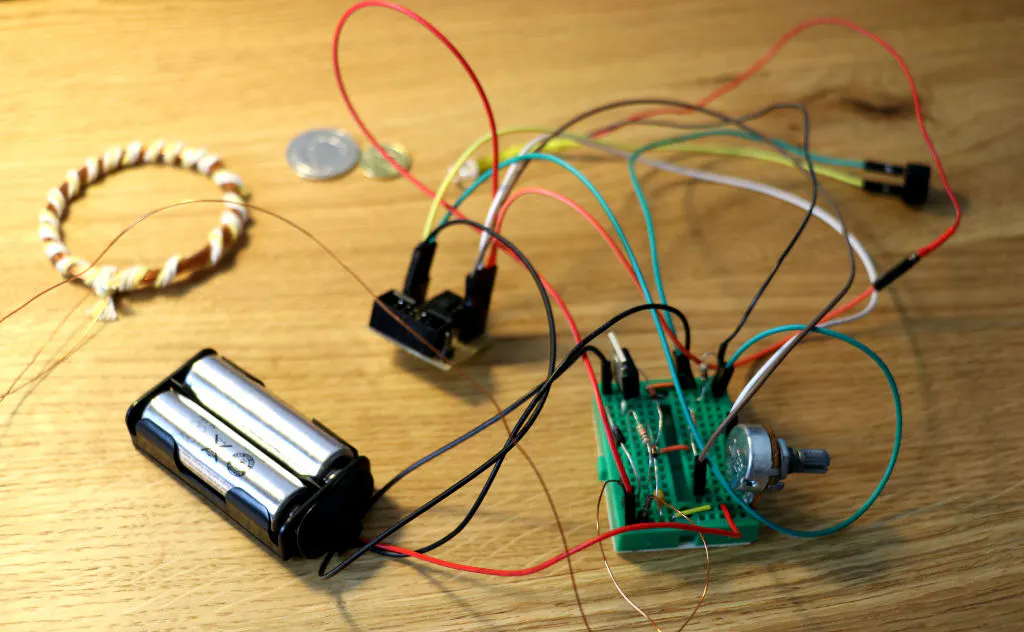## Things used in this project

### Hardware components

 Microchip ATtiny13
×1Resistor 10k ohm
×2
 IRF3205
×1LED (generic)
×1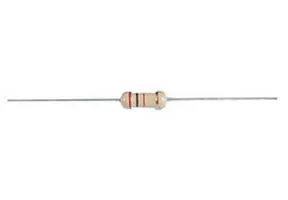Resistor 220 ohm
×2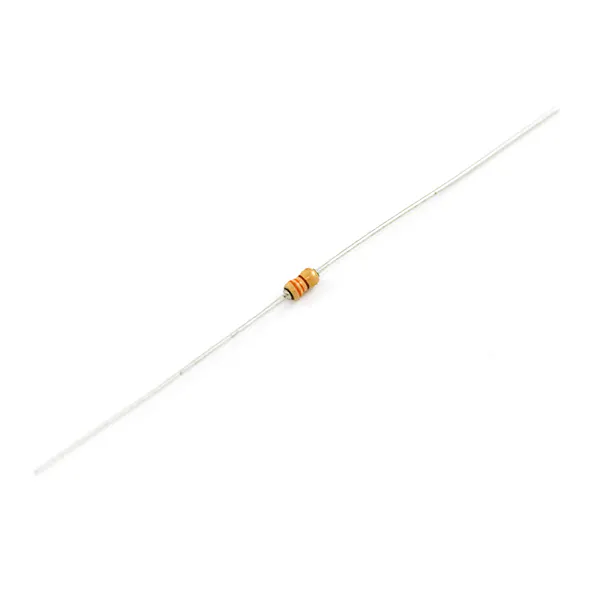Resistor 330 ohm
×1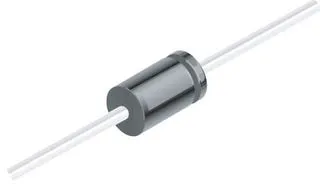1N4007 – High Voltage, High Current Rated Diode
×1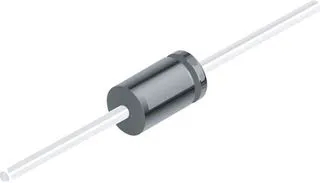1N4148 – General Purpose Fast Switching
×1
 capacitor 470nF
×1
 variable resistor 10kΩ
×1
 Coil ∅ 50-55mm (about 30 turns of 0.5 DNE)
×1

## Schematics

### Schematic of ATtiny13 PI metal detector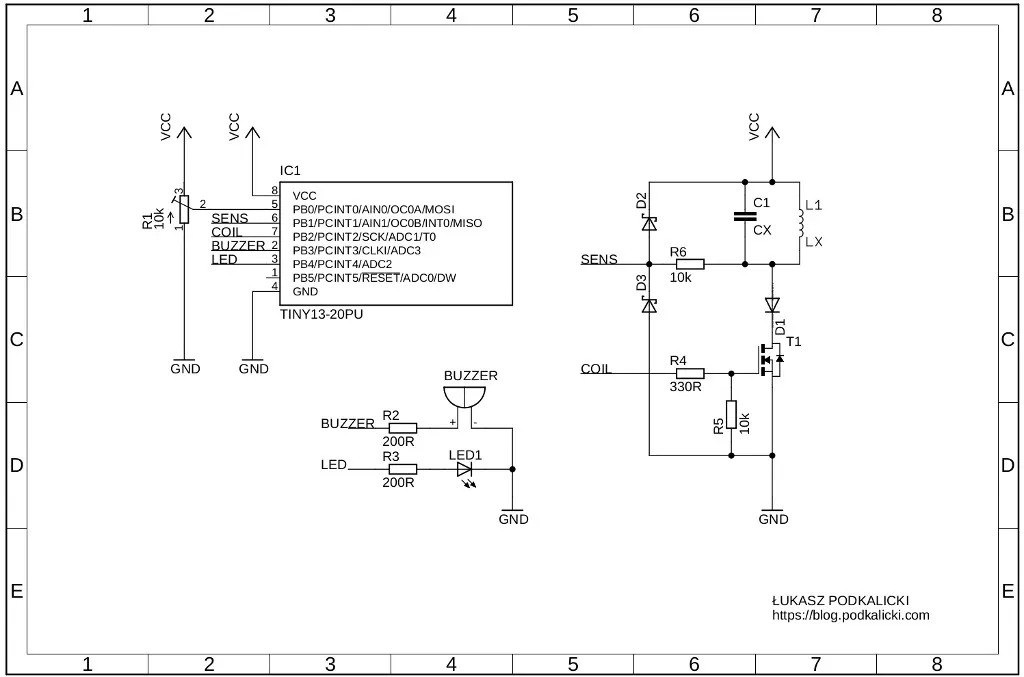## Code

### Code snippet #2

Plain text
```/**
* Copyright (c) 2019, Łukasz Marcin Podkalicki <lpodkalicki@gmail.com>
* ATtiny13/037
* Example of simple PI (Pulse Induction) metal detector.
*/

#include <avr/io.h>
#include <util/delay.h>
#include <avr/interrupt.h>

#define	COIL_PIN                  PB2
#define	BUZZER_PIN                PB3
#define	LED_PIN                   PB4

#define	PULSE_WIDTH               (32) // microseconds
#define	CALIBRATION_ATTEMPTS_MAX  (128)
#define	MEASUREMENT_ATTEMPTS_MAX  (2048)

#define	SIGNAL_ON()               (PORTB |= _BV(LED_PIN)|_BV(BUZZER_PIN))
#define	SIGNAL_OFF()              (PORTB &= ~(_BV(LED_PIN)|_BV(BUZZER_PIN)))

static uint16_t
measure_decay(void)
{
uint16_t i, counter = 0, decay = 0;

PORTB |= _BV(COIL_PIN); // pulse on
_delay_us(PULSE_WIDTH); // pulse delay
PORTB &= ~_BV(COIL_PIN); // pulse off

for (i = 0; i < MEASUREMENT_ATTEMPTS_MAX; ++i) {
if (ACSR & _BV(ACO)) {
decay = counter;
}
counter++;
}

return decay;
}

static uint16_t
calibration(void)
{
uint8_t i;
uint16_t tmp, decay = 0;

/* calibration process */
for (i = 0; i < CALIBRATION_ATTEMPTS_MAX; ++i) {
tmp = measure_decay();
if (tmp > decay) {
decay = tmp;
}
}

/* signalize end of calibration */
for (i = 0; i < 3; ++i) {
for (tmp = 0; tmp < 64; ++tmp) {
SIGNAL_ON();
_delay_ms(0.3);
SIGNAL_OFF();
_delay_ms(0.3);
}
_delay_ms(64);
}

return decay;
}

int
main(void)
{
uint16_t decay_cur, decay_max;

/* setup */
DDRB = _BV(COIL_PIN)|_BV(LED_PIN)|_BV(BUZZER_PIN); // set COIL, LED and BUZZER pins as output
ACSR = 0; // clear register

decay_max = calibration() - 1;
_delay_ms(500);

/* loop */
while (1) {
decay_cur = measure_decay();
if (decay_cur < decay_max) {
SIGNAL_ON();
_delay_us(100);
}
SIGNAL_OFF();
}
}
```

## Credits

### Łukasz Podkalicki

1 project • 0 followers